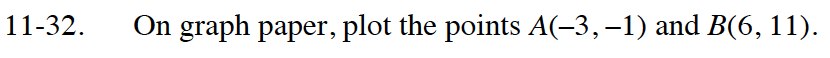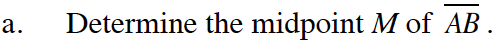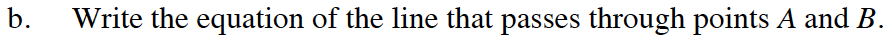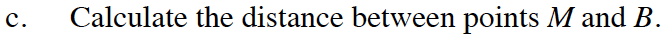### Home > INT1 > Chapter Ch11 > Lesson 11.1.3 > Problem11-32

11-32.
1. On graph paper, plot the points A(−3, −1) and B(6, 11). 11-32 HW eTool (Desmos). Homework Help ✎

1. Determine the midpoint M of AB.

2. Write the equation of the line that passes through points A and B.

3. Calculate the distance between points M and B.What is the y-intercept?
What is the slope?Use a slope triangle drawn between M and P, and the Pythagorean Theorem to find the distance.

Use the eTool below to solve each part of the problem.
Click the link at the right to view full version of the eTool: Int1 11-32 HW eTool.